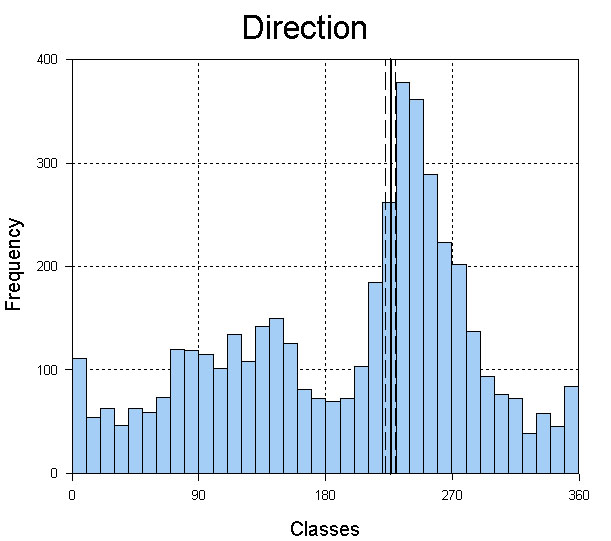# Oriana Graphics

• Graph types include
• Rose diagrams
• Circular histograms
• Linear histograms
• Arrow graphs – like a histogram, but an arrow is used for each class rather than a bar or wedge
• Raw data plots
• Distribution graphs – plot data against expected distribution for either uniform or von Mises distributions.
• Stacked and stepped circular histograms/rose diagrams- bars or wedges divided into segments to reflect the proportions of each subgroup within the data.
• Stacked raw data plots – points representing each data point are colored according to their subgroup
• Two-variable circular histograms – segments of each bar represents the classes of a second circular or linear variable (e.g. wind speed plotted as segments on bars representing wind direction)
• Circular-linear plots – these incorporate linear data along with your circular data. An arrow, bar or wedge is drawn for each case, with the length representing the linear data.
• Circular scatterplots – these also plot linear and circular data, with each data pair being plotted as points on a circular plot
• X/Y Scatterplots – can plot any circular or linear variable on X/Y Cartesian axes.
• Q-Q plot – compare distributions of two circular samples to assess whether they have the same distribution
• Mean and confidence limits can be shown on histograms. Mean can alternatively be represented by a mean vector, where the length of the arrow reflects the mean vector length (r).
• Circular graphs can have a circle showing the critical value of the Rayleigh test. The length of the r vector can then be compared to this circle to assess its significance.
• With paired vector data (consisting of angle and length) the mean vector can be weighted by the vector lengths.
• Axes can be extensively customized with regard to number of grid lines, scaling (linear, percentage or logarithmic), format and orientation of labels.
• Circular graphs can be drawn with the 0°/N point at user chosen position on the circle, and oriented either clockwise or counter-clockwise.
• Doubled-angle plots, which plot only the data between 0-180°, but spread around the full circumference of the circle, can be produced. This type of graph is used in fields such as ophthalmology.
• Circular-linear plots can have a standard deviation ellipse plotted (also used in opthalmology).
• Division of segments in two-variable histograms can be customized or manually defined.
• Bar width of histograms and rose diagrams can be changed.
• Rose diagrams can have frequency depicted by either radius or area of wedge.
• Linear histograms can be plotted as double width plots to emphasise cyclicity in data.
• Table of frequencies used to produce histograms can be displayed in the Results window.
• Graph types and options can be modified without having to create a new graph window.
• Graph appearance can be customized (fonts, color of axes, thickness of lines and grids, color of background of various sections, series of colors, patterns and symbols for stacked and subgrouped diagrams).